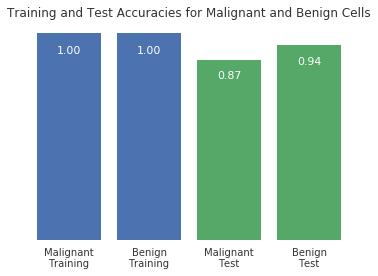# Predicting Cancer (Course 3, Assignment 1)

This assignment uses the Breast Cancer Wisconsin (Diagnostic) Database to create a classifier that can help diagnose patients.

## Imports

import numpy
import pandas


## The data

cancer = load_breast_cancer()


This data set has 569 rows (cases) with 30 numeric features. The outcomes are either 1 - malignant, or 0 - benign.

From their description:

Features are computed from a digitized image of a fine needle aspirate (FNA) of a breast mass.  They describe characteristics of the cell nuclei present in the image.


The object returned by load_breast_cancer() is a scikit-learn Bunch object, which is similar to a dictionary, but like pandas, also supports using dot-notation to retrieve attributes when possible (i.e. no spaces in the keys).

print(cancer.keys())

dict_keys(['DESCR', 'target', 'feature_names', 'data', 'target_names'])


## Question 0 (Example)

How many features does the breast cancer dataset have?

def answer_zero():
"""number of feature names in the data

Returns:
int: count of feature names in the 'cancer' data-set
"""
return len(cancer['feature_names'])

answer_zero()

30


## Question 1

Scikit-learn works with lists, numpy arrays, scipy-sparse matrices, and pandas DataFrames, so converting the dataset to a DataFrame is not necessary for training this model. Using a DataFrame does however help make many things easier such as munging data, so let's practice creating a classifier with a pandas DataFrame.

def answer_one():
"""converts the sklearn 'cancer' bunch

Returns:
pandas.DataFrame: cancer data
"""
data = numpy.c_[cancer.data, cancer.target]
columns = numpy.append(cancer.feature_names, ["target"])
return pandas.DataFrame(data, columns=columns)

frame = answer_one()
assert frame.shape == (len(cancer.target), 31)


## Question 2

What is the class distribution? (i.e. how many instances of malignant and how many benign?)

def answer_two():
"""calculates number of malignent and benign

Returns:
pandas.Series: counts of each
"""
counts = cancerdf.target.value_counts(ascending=True)
counts.index = "malignant benign".split()
return counts

output = answer_two()
assert output.malignant == 212
assert output.benign == 357


## Question 3

Split the DataFrame into X (the data) and y (the labels).

def answer_three():
"""splits the data into data and labels

Returns:
(pandas.DataFrame, pandas.Series): data, labels
"""
X = cancerdf[cancerdf.columns[:-1]]
y = cancerdf.target
return X, y

x, y = answer_three()
assert x.shape == (569, 30)
assert y.shape == (569,)


## Question 4

Using train_test_split(), split X and y into training and test sets (X_train, X_test, y_train, and y_test).

from sklearn.model_selection import train_test_split

"""splits data into training and testing sets

Returns:
tuple(pandas.DataFrame): x_train, y_train, x_test, y_test
"""
return train_test_split(X, y, train_size=426, test_size=143, random_state=0)

x_train, x_test, y_train, y_test = answer_four()
assert x_train.shape == (426, 30)
assert x_test.shape == (143, 30)
assert y_train.shape == (426,)
assert y_test.shape == (143,)


## Question 5

Using KNeighborsClassifier, fit a k-nearest neighbors (knn) classifier with X_train, y_train and using one nearest neighbor (n_neighbors = 1).

from sklearn.neighbors import KNeighborsClassifier

"""Fits a KNN-1 model to the data

Returns:
sklearn.neighbors.KNeighborsClassifier: trained data
"""
X_train, X_test, y_train, y_test = answer_four()
model = KNeighborsClassifier(n_neighbors=1)
model.fit(X_train, y_train)
return model

knn = answer_five()
assert type(knn) == KNeighborsClassifier
assert knn.n_neighbors == 1


## Question 6

Using your knn classifier, predict the class label using the mean value for each feature.

You can use cancerdf.mean()[:-1].values.reshape(1, -1) which gets the mean value for each feature, ignores the target column, and reshapes the data from 1 dimension to 2 (necessary for the predict method of KNeighborsClassifier).

def answer_six():
"""Predicts the class labels for the means of all features

Returns:
numpy.array: prediction (0 or 1)
"""
means = cancerdf.mean()[:-1].values.reshape(1, -1)
return model.predict(means)

answer_six()

array([ 1.])


## Question 7

Using your knn classifier, predict the class labels for the test set X_test.

def answer_seven():
"""predicts likelihood of cancer for test set

Returns:
numpy.array: vector of predictions
"""
X_train, X_test, y_train, y_test = answer_four()
return knn.predict(X_test)

predictions = answer_seven()
assert predictions.shape == (143,)
assert set(predictions) == {0.0, 1.0}

print("no cancer: {0}".format(len(predictions[predictions==0])))
print("cancer: {0}".format(len(predictions[predictions==1])))


## Question 8

Find the score (mean accuracy) of your knn classifier using X_test and y_test.

def answer_eight():
"""calculates the mean accuracy of the KNN model

Returns:
float: mean accuracy of the model predicting cancer
"""
X_train, X_test, y_train, y_test = answer_four()
return knn.score(X_test, y_test)

answer_eight()


## Optional plot

Try using the plotting function below to visualize the differet predicition scores between training and test sets, as well as malignant and benign cells.

%matplotlib inline

def accuracy_plot():
import matplotlib.pyplot as plt

X_train, X_test, y_train, y_test = answer_four()

# Find the training and testing accuracies by target value (i.e. malignant, benign)
mal_train_X = X_train[y_train==0]
mal_train_y = y_train[y_train==0]
ben_train_X = X_train[y_train==1]
ben_train_y = y_train[y_train==1]

mal_test_X = X_test[y_test==0]
mal_test_y = y_test[y_test==0]
ben_test_X = X_test[y_test==1]
ben_test_y = y_test[y_test==1]

scores = [knn.score(mal_train_X, mal_train_y), knn.score(ben_train_X, ben_train_y),
knn.score(mal_test_X, mal_test_y), knn.score(ben_test_X, ben_test_y)]

plt.figure()

# Plot the scores as a bar chart
bars = plt.bar(numpy.arange(4), scores, color=['#4c72b0','#4c72b0','#55a868','#55a868'])

# directly label the score onto the bars
for bar in bars:
height = bar.get_height()
plt.gca().text(bar.get_x() + bar.get_width()/2, height*.90, '{0:.{1}f}'.format(height, 2),
ha='center', color='w', fontsize=11)

# remove all the ticks (both axes), and tick labels on the Y axis
plt.tick_params(top='off', bottom='off', left='off', right='off', labelleft='off', labelbottom='on')

# remove the frame of the chart
for spine in plt.gca().spines.values():
spine.set_visible(False)

plt.xticks([0,1,2,3], ['Malignant\nTraining', 'Benign\nTraining', 'Malignant\nTest', 'Benign\nTest'], alpha=0.8);
plt.title('Training and Test Accuracies for Malignant and Benign Cells', alpha=0.8)
accuracy_plot()